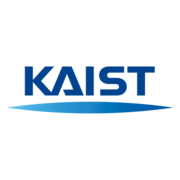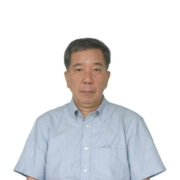# 6-7 Homogeneous linear equations with constant coefficients

Loading...From the course by Korea Advanced Institute of Science and Technology(KAIST)
Introduction to Ordinary Differential Equations
196 ratingsKorea Advanced Institute of Science and Technology(KAIST)

### Introduction to Ordinary Differential Equations

196 ratings
From the lesson
Linear Second order equations 2

#### Meet the Instructors

•Kwon, Kil Hyun
Professor
Department of Mathematical Sciences